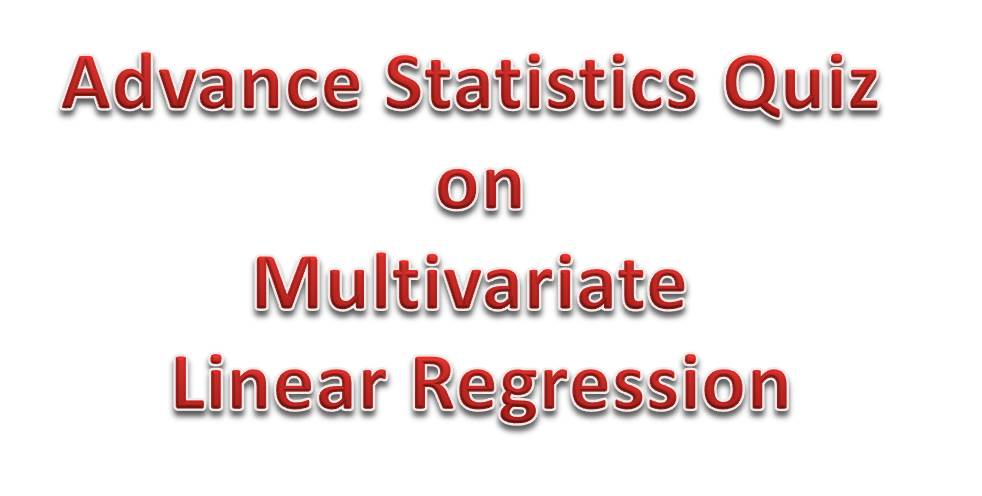# Advanced Statistics Quiz 8a – Concepts from Multivariate Linear RegressionHello, good to see you! I made this post to help you prepare for oral and written quiz or exam on advanced or mathematical statistics as a continuation of the Advanced Statistics Quiz 8
Question 1: Outline and explain the effects of Multicollinearity?
The effects of multicollinearity are:
• Variation Inflation Factor(VIF)
• Tolerance
• Condition Indices
Variation Inflation Factor(VIF): The variation inflation factor is a metric used to measure the severity of multicolinearity. It is the ration of the variation of a model w.r.t mutiple terms divided by the variance of the model with one term alone. It explains how much the variance of an estimated regression coefficient is changed due to multicollinearity.
Tolerance: This is the inversion of VIF and indicates that a variable under consideration is almost a perfect linear combination of the independent variables already in the equation and need not be added to the regression equation.Tolerance values of the range of 0.2 and below is considered good.
Condition Indices: The condition indices is a function of eigenvalues and measures the relative amount of variation associated with an eigenvalue such that a large condition index indicates a high degree of collinearity.
Question 2: What is Leverage Matrix in Multivariate Linear Regression?
The leverage matrix which is also called the hat matrix or projection matrix is used for investigating whether one or more observations are outlying with regards to their X-values and therefore excessively influencing the results of the regression
Question 3: What is Binary Logistic Regression
This is a regression model where the dependent variable is binary or categorical and is used to determine the the probability of a binary response based on the independent variables or feature vector.
We continues in the next part.
Thank you!